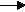gcsescience.com                                       21                                       gcsescience.com

Moles

Volumes of Gases.

Example 2.

What volume of carbon dioxide is produced
by burning 90 g  of ethane in air?

Method.
1) Find how many moles of ethane are present in 90 g  of ethane.
RAM of  C = 12RAM of  H = 1.

RMM of ethane (C2H6)  =  (2 x 12) + (6 x 1)
= 30.

moles = mass ÷ RAM

moles = 90 ÷ 30

= 3·0 moles of ethane.

2) Write the equation for the reaction.

ethane    oxygencarbon dioxide + water.
2C2H6(g)  + 7O2(g)4CO2(g)   +   6H2O(l)

Use the big numbers to find the proportion of reactant to product.
2C2H6 makes 4CO2, the proportion is 2 to 4,  or 1 to 2,
so 3·0 moles of ethane will make 6·0 moles of carbon dioxide.

3) Convert moles into volume.

volume moles x 24,000 cm3

volume 6·0 x 24,000 cm3
= 144,000 cm3 of carbon dioxide.

So, burning 90 g  of ethane in air
will produce 144,000 cm3 of carbon dioxide.

gcsescience.com       The Periodic Table       Index       Moles Quiz       gcsescience.com